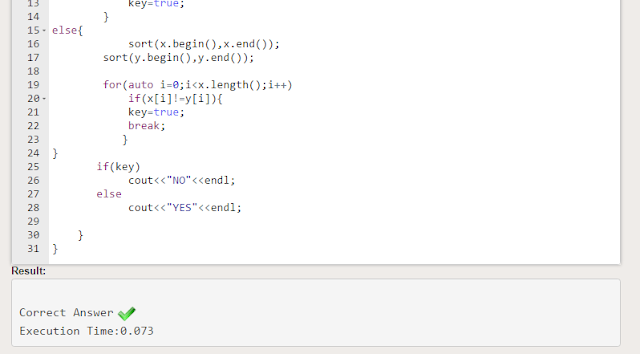# Anagram Geeks for Geeks Solution in C++ with Output

Given two strings a and b consisting of lowercase characters. The task is to check whether two given strings are an anagram of each other or not. An anagram of a string is another string that contains the same characters, only the order of characters can be different. For example, act and tac are an anagram of each other.

Note: If the strings are anagrams you have to return True or else return False

Example 1:

```Input:a = geeksforgeeks, b = forgeeksgeeks
Output: YES
Explanation: Both the string have same characters with
same frequency. So, both are anagrams.```

Example 2:

```Input:a = allergy, b = allergic
Output: NO
Explanation: Characters in both the strings are
not same, so they are not anagrams.```

You don't need to read input or print anything. Your task is to complete the function isAnagram() which takes the string a and string b as input parameter and check if the two strings are an anagram of each other. The function returns true if the strings are anagrams else it returns false.

Note: In python, you have to return True or False.

Expected Time Complexity:O(|a|+|b|).
Expected Auxiliary Space: O(Number of distinct characters).

Note: |s| represents the length of string s.

Constraints:
1 ≤ |a|,|b| ≤ 105

## Anagram Geeks for Geeks Solution in C++

``````#include <bits/stdc++.h>
using namespace std;

int main() {
int t;
cin>>t;
while(t--){
string x,y;
cin>>x>>y;
auto key=false;
if(x.length()<y.length()||y.length()<x.length())
{
key=true;
}
else{
sort(x.begin(),x.end());
sort(y.begin(),y.end());

for(auto i=0;i<x.length();i++)
if(x[i]!=y[i]){
key=true;
break;
}
}
if(key)
cout<<"NO"<<endl;
else
cout<<"YES"<<endl;

}
}``````

### The Output of Anagram Geeksforgeeks SolutionPrevious Post
Next Post

#### post written by: Ghanendra Yadav

Hi, I’m Ghanendra Yadav, SEO Expert, Professional Blogger, Programmer, and UI Developer. Get a Solution of More Than 500+ Programming Problems, and Practice All Programs in C, C++, and Java Languages. Get a Competitive Website Solution also Ie. Hackerrank Solutions and Geeksforgeeks Solutions. If You Are Interested to Learn a C Programming Language and You Don't Have Experience in Any Programming, You Should Start with a C Programming Language, Read: List of Format Specifiers in C.